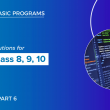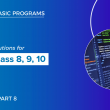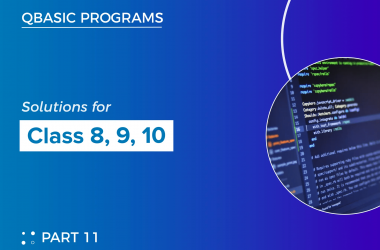# Qbasic programs solutions(part 7) for class 8, class 9 and class 10

Here, I have listed some of the qbasic programs. We also have mobile app where you can view all the qbasic programs. For better experience I highy recommend you to use our mobile app

121   Write a program in qbasic to generate the series 55555   5555   555   55   5

``````CLS
A = 55555
FOR I = 1 TO 5
PRINT A;
A = A \ 10
NEXT I
END ``````

122  Write a program in qbasic to generate the series 1   2   3   5…………9th terms

``````CLS
A = 1
B = 2
FOR I = 1 TO 9
PRINT A;
C = A + B
A = B
B = C
NEXT I
END ``````

123  Write a program in qbasic to print numbers 70   61   52…..7

``````CLS
FOR I = 70 TO 7 STEP -9
PRINT I
NEXT I
END``````

124  Write a program in qbasic to generate the series 100   95   90…………………10th term

``````CLS
A = 100
FOR I = 1 TO 10
PRINT A;
A = A - 5
NEXT I
END ``````

125  Write a program in qbasic TO ENTER ANY DIGIT AND PRINT ITS REVERSED FORM

``````CLS
INPUT "ENTER ANY NUMBER"; N
S = 0
WHILE N  <> 0
R = N MOD 10
S = S * 10 + R
N = N \ 10
WEND
PRINT "REVERSED DIGITS="; S
END ``````

126  Write a program to enter any three numbers and display the smallest one.

``````CLS
INPUT “ENTER ANY THREE NUMBERS”; A, B, C
IF A < B AND A < C THEN
PRINT A; “IS SMALLEST”
ELSEIF B < A AND B < C THEN
PRINT B; “IS SMALLEST”
ELSE
PRINT C; “IS SMALLEST”
END IF
END ``````

127  Write a program in qbasic to print the sum of square of odd numbers up to 100

``````CLS
FOR I = 1 TO 100 STEP 2
S = S + I ^ 2
NEXT I
PRINT “SUM OF SQUARE OF ODD NUMBERS UP TO 100” ; S
END ``````

128  Write a program in qbasic to print the sum of cube of even numbers between 2 to 50

``````CLS
FOR I = 2 TO 50 STEP 2
S = S + I
NEXT I
PRINT “SUM OF EVEN NUMBERS BETWEEN 2 TO 50” ; S
END
﻿``````

129  Write a program to input a mark in a subject of a student and check if the student is pass or nor.

``````CLS
INPUT”ENTER THE MARKS IN ANY SUBJECT”;A
IF A>40 THEN
PRINT” YOU ARE PASS”
ELSE
PRINT”YOU ARE FAIL”
END ``````

130  Write a program in qbasic to ask number and find product of digits

``````INPUT "ENTER ANY NUMBER"; N
P = 1
WHILE N  < > 0
R = N MOD 10
P = P * R
N = N \ 10
WEND
PRINT "PRODUCT OF DIGITS"; P
END ``````

131  Write a program in qbasic using to print first 13 odd numbers

``````CLS
A = 1
FOR I = 1 TO 13
PRINT A,
A = A+ 2
NEXT I
END``````

132  Write a program in qbasic to ask any number and find sum of factors

``````CLS
INPUT "ENTER ANY NUMBER"; N
S = 0
FOR I = 1 TO N
IF N MOD I = 0 THEN S = S + I
NEXT I
PRINT "SUM OF FACTORS="; S
END ``````

133  Write a program in qbasic to enter any ten strings and sort in descending order

``````CLS
DIM N(10) AS STRING
FOR I = 1 TO 10
INPUT "ENTER THE STRINGS"; N(I)
NEXT I
FOR I = 1 TO 10
FOR J = 1 TO 10 - I
IF N(J)  <  N(J + 1) THEN SWAP N(J), N(J + 1)
NEXT J
NEXT I
PRINT "STRINGS ARRANGED IN DESCENDING ORDER"
FOR I = 1 TO 10
PRINT N(I)
NEXT I
END ``````

134  Write a program in qbasic to ask any string and print only vowels

``````CLS
INPUT "ENTER ANY STRING"; S\$
FOR I = 1 TO LEN(S\$)
B\$ = MID\$(S\$, I, 1)
C\$ = UCASE\$(B\$)
IF C\$ = "A" OR C\$ = "E" OR C\$ = "I" OR C\$ = "O" OR C\$ = "U" THEN
PRINT B\$
END IF
NEXT I
END
﻿``````

135  Write a program in qbasic to ask any number and print the factorial of a given number

``````CLS
INPUT "ENTER ANY NUMBER"; N
F = 1
FOR I = 1 TO N
F = F * I
NEXT I
PRINT "FACTORIAL ="; F
END ``````

136  Write a program in qbasic to print square of all numbers from 1 to 50

``````CLS
FOR I = 1 TO 50
PRINT I ^ 2,
NEXT I
END
﻿``````

137   Write a program in qbasic to print all prime numbers from 1 to 100

``````CLS
FOR N = 1 TO 100
C = 0
FOR I = 1 TO N
IF N MOD I = 0 THEN C = C + 1
NEXT I
IF C = 2 THEN PRINT N,
NEXT N
END ``````

138  Write a program in qbasic to ask number and find sum of square of even digits

``````
CLS
INPUT "ENTER ANY NUMBER"; N
S = 0
WHILE N < > 0
R = N MOD 10
IF R MOD 2 = 0 THEN S = S + R ^ 2
N = N \\ 10
WEND
PRINT "SUM OF SQUARE OF EVEN DIGITS"; S
END ``````

139   Write a program in qbasic to ask number and find sum of square of odd digits

``````CLS
INPUT "ENTER ANY NUMBER"; N
PRINT "SQUARE OF ODD DIGITS ARE ";
WHILE N <> 0
R = N MOD 10
IF R MOD 2 = 1 THEN PRINT R ^ 2;
N = N \ 10
WEND
END ``````

140  Write a program in qbasic to ask number and find product of even digits

``````CLS
INPUT "ENTER ANY NUMBER"; N
P = 1
WHILE N <> 0
R = N MOD 10
IF R MOD 2 = 0 THEN P = P * R
N = N \ 10
WEND
PRINT "PRODUCT OF EVEN DIGITS"; P
END ``````

##### Previous Post## Qbasic programs solutions(part 6) for class 8, class 9 and class 10

##### Next Post## Qbasic programs solutions(part 8) for class 8, class 9 and class 10

##### Related Posts## Debugging in QBASIC

Debugging is a crucial skill for programmers, even for those using QBASIC, a popular language for beginners. As…## Exploring the Magic of Boolean Logic in QBasic Programming

In the world of programming, understanding Boolean logic is like deciphering a secret code that unlocks a realm…## Qbasic programs solutions(part 11) for class 8, class 9 and class 10

Here, I have listed some of the qbasic programs. We also have mobile app where you can view…